SSC BOARD PAPERS IMPORTANT TOPICS COVERED FOR BOARD EXAM 2024

### Question 5. Solve the following simultaneous equation graphically. 3x – y = 2; 2x – y = 3

Question 5.

Solve the following simultaneous equation graphically.

3x – y = 2; 2x – y = 3

Eq. I =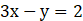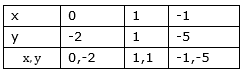Eq. II =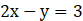Calculating intersecting point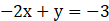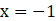Putting x= –1 in Eq.I

3× –1 – y = 2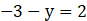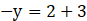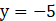Intersection point (–1,–5)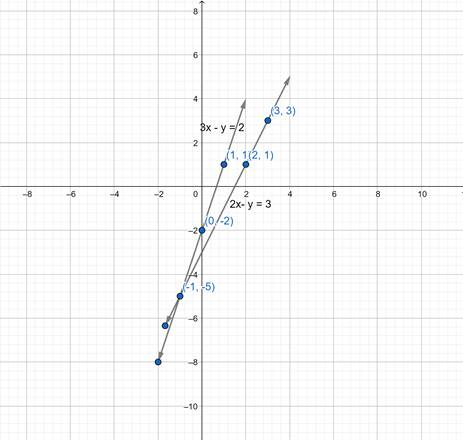MATHS I SSC NEW SYLLABUS

Chapter No. 1. Linear Equations in Two Variables

Practice Set 1.1

Practice Set 1.2

Practice Set 1.3

Practice Set 1.4

Practice Set 1.5

Problem Set No. 1

Practice Set 2.1

Practice Set 2.2

Practice Set 2.3

Practice Set 2.4

Practice Set 2.5

Practice Set 2.6

Problem Set No. 2

Chapter No. 3: Arithmetic Progression

Practice Set 3.1

Practice Set 3.2

Practice Set 3.3

Practice Set 3.4

Problem Set No 3

Chapter 4: Financial Planning.

Practice set 4.1

Practice set 4.2

Practice set 4.3

Practice set 4.4

Problem set 4 A

Problem set 4 B

CHAPTER NO. 5. PROBABILITY

PRACTICE SET 5.1

PRACTICE SET 5.4

PROBLEM SET NO. 5

Chapter No. 6: STATISTICS

PRACTICE SET 6.1

PRACTICE SET 6.2

PRACTICE SET 6.3

PRACTICE SET 6.4

PRACTICE SET 6.5

PRACTICE SET 6.6

PROBLEM SET NO. 6

BOARD PAPERS WITH COMPLETE SOLUTION

Mathematics Part - i Paper Pattern and Guidelines

SSC Maths I March 2019 Board Paper Solution 10th Standard 11th, March, 2019

GENERAL MATHS ALGEBRA BOARD PAPERS

MATHS I PRACTICE PAPER FOR SCHOOL PRELIMINARY EXAM. [AS PER NEW SYLLABUS]

Paper No. 1

Paper No. 2

Paper No. 3

ALGEBRA SSC OLD SYLLABUS

ARITHMETIC PROGRESSION

LINEAR EQUATIONS

PROBABILITY

STATISTICS ONE

STATISTICS TWO

EXTRA HOTS SUMS

FORMULA

ALGEBRAIC FORMULAE